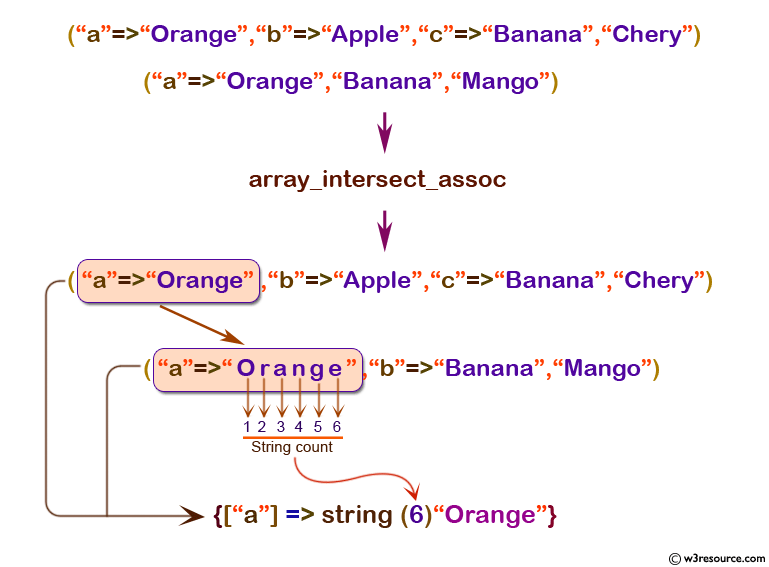# PHP: array_intersect_ assoc() function

## PHP: Computes the intersection of arrays with additional index check

The array_intersect_assoc() is used to create an array containing keys and values of the first array whose values (i.e. from the first array) are present in all other arrays, while index of values is same for all the given arrays.

Version:

(PHP 4 and above)

Syntax:

`array_intersect_assoc(array1, array2, .. )`

Parameters:

Name Description Required /
Optional
Type
array1 The first array. Required Array
array2 An array to check values against the first array. Required Array

Return value:

An associative array containing all the values in array1 that are present in all of the arguments.

Value Type: Array

Example :

``````<?php
\$array1 = array("a" => "Orange", "b" => "Apple", "c" => "Banana", "Chery");
\$array2 = array("a" =>"Orange", "Banana","Mango");
\$result = var_dump(array_intersect_assoc(\$array1, \$array2));
print_r(\$result)
?>
``````

Output:

`array(1){ ["a"]=> string(6) "Orange"} `

Pictorial Presentation:View the example in the browser

Practice here online:

PHP Function Reference

Previous:array_flip
Next: array_intersect_ key

﻿

## PHP: Tips of the Day

Filters the collection using the given callback

Example:

```<?php
function tips_reject(\$items, \$func)
{
return array_values(array_diff(\$items, array_filter(\$items, \$func)));
}

print_r(tips_reject(['Apple', 'Pear', 'Kiwi', 'Banana'], function (\$item) {
return strlen(\$item) > 4;
}));
?>
```

Output:

```Array
(
 => Pear
 => Kiwi
)
```